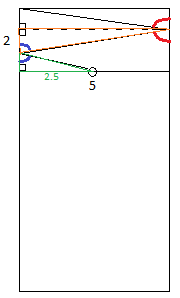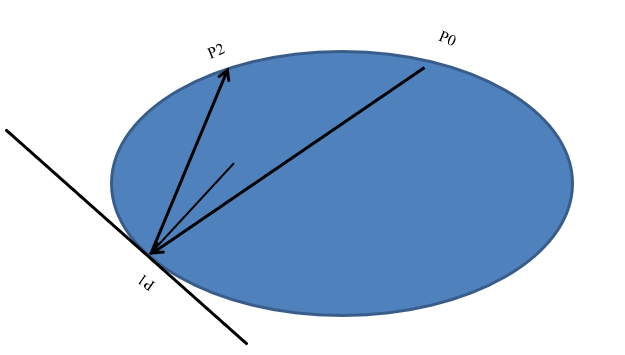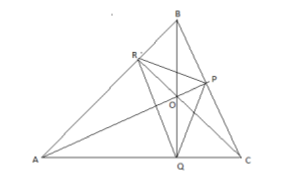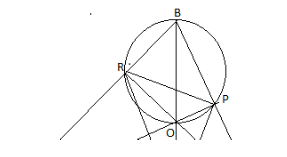Aug 21, 2020 in

# Use of Math in Life

## Introduction

Mathematics is everywhere and we use it in our day to day undertakings. We use mathematics in counting by use of addition- subtraction and multiplication-division arithmetic. The Fobinacci sequence which involves deriving a number from addition of the two previous numbers has been applied in nature to determine the patterns of flower in pineapples, sunflowers, and others. Generally, everything in the world has mathematics in it; hence math is life.

Billiards is the most mathematically important sport in history. Mathematicians have attempted to learn Billiards for ages. In this essay, I will discuss various mathematical aspects relating to billiards and how various shapes of billiard tables affect the gameplay of billiards. There are various types of billiards and every type has a distinctive mathematical approach.

Type of assignment
Writer level
Urgency
Number of pages
Total price:

### Rectangular Billiards

In mathematical billiards, it is important to note that the angle of incidence is equivalent to the angle of reflection. This fact aids to substantiate formulae and comprehend paths of billiards balls. Mathematicians’ focus on bounds of the billiard ball and the theory that the angle of reflection is equal to the angle of incidence helps them know the angle the ball jumps off at. For example, given that a table has a width of 5ft and the billiard ball is 2ft from the top you may be asked to show the point where a ball ought to strike the length of a table so that a two-cushion bank shot shall put the ball along a path that finishes at the upper left bend. To find a solution to this problem on should consider the characteristics of cosine, sine and tangent and the fact that incidence angle and angle of reflection have the same value. We are required to create two right angled triangles by intersecting a line on the second jump of the ball, which creates a right angle with the left side of the table (See figure A).Figure A: billiard ball shot hits the top left angle once it bounds off 2 of the cushions.

We realize that both the orange and green triangle share similar angles from the first jump. Due to the fact that the reflection angle and the incidence angle are analogic to the right angles in these triangles, the third angles must be equal because all the angles of a triangle must amount to 180 degrees. The AAA triangular property which stands for” angle, angle, angle,” ascertains that these two triangles are analogous. Where we consider width, we recognize that the base of the smaller triangle is 2.5ft and the larger triangle is 5ft transversely. From this we can infer that the large triangle is two times bigger than the smaller one since one side of the smaller triangle is half the size of the corresponding side of the larger triangle but all angles share equal values. With the base of the smaller triangle as X, the larger triangle which is double in size has the base as 2X. The base of the third triangle is denoted as 2X since it is intersected from two larger triangles. From these details, we get the equation, 2X +2X+X=2 hence X=2∕5. After calculating the value of X, we can now determine the base of the two larger triangles. (Note that they have identical angles) 2 2∕5 +2 2∕5 = 8∕5= 1 3∕5. This determines the answer to the problem since we now know that the ball has to strike the length of the billiards table 1 3∕5ft from the top of the table.

### Elliptical billiards

The study on billiards mathematics has perpetuated far beyond the rectangular tables. Mathematicians have developed theorems and approaches for billiards on non- rectangular tables. The ellipse is one of the shapes I have focused on. Similar to rectangular tables, elliptical billiards follow the principle of path of a billiard ball. Elliptical billiards consists of different path patterns that a ball can follow. The trajectory of a billiard ball is described by a line segment and a sequence of positions denoted as p0, p1, p2, p3... The starting position of a billiard p0 is constantly at the side of the elliptical table with other positions, as the points where the ball is reflected off the edge of the table. If a path hits an edge and deflects back to the starting point, then it is known to be an n-cycle. Through various theorems we are able to answer questions relating to billiard balls and the path. For example, we can be able to know the number of time a ball can bounce before it returns to the starting position. One can also determine how many n-cycles a give elliptical billiard table has. While studying elliptical billiards, it is essential to underscore the fact that the incidence angle is similar to reflection angle. When we compare rectangular and elliptical billiards, we recognize that the incidence and reflection angles are formed at the edge of the table; however, the angle of incidence in elliptical billiards is created at the tangent line to the ellipse at that point. Observing at Figure B, we realize that the angle made by the line p0p1 is equivalent to angle made by line p1p2. P1 illustrates a line identified as normal line which is perpendicular to a bisecting line known as the tangent line.Figure B

P0, P1, P2 shows the trajectory of the billiard ball

The angle made with the tangent line.

The normal line - P1

### Circular Billiards

A circle is another geometric shape that has been intensively studied by mathematician over the years just like ellipse. The rotational symmetry is one of the properties of a circle that is very instrumental in studies of circular billiards paths. Moreover, the rule of the incidence angle equals reflection angle applies. Hence, after every reflection in a circle, the angle remains constant. Due to identical curvature at every point, when a circle is rotated, it looks the same unlike ellipse which has different arc angle different points. As a result, each position creates a different angle for the billiard ball to bounce at which translates to formation of patterns in the trajectories of billiards balls.In a circle, the reflection angle is represented by α while the path of a billiard ball is designated by n-periodic. Where n is positive integer, for every integer p˂ n∕ 2 , where p is comparatively prime to n, there is a distinctive n-periodic trajectory. A circle consists of two types of 5-cycle trajectories, the pentagram where p=2 and a pentagon where p=1 (see figure C and D). This means that shooting a billiard ball could result to it returning to its starting point in two distinct versions. Furthermore, 2- cycles trajectories in a circle are endless. It is certainly correct to argue that a ball would return to its starting position if it is shot from the center of a circle. This is because of the rotational symmetry in a circle. There are instances when an n-periodic orbit is formed whereby, n is an integer and when the ball is shot, it shall return to the starting point forming an n-periodic table. This phenomenon occurs due the fact that n is rational. Some integers like 5 can configure numerous n-periodic orbits.

### Triangular Billiards

I also took keen interest in mathematical billiards in polygons with the triangle being the main focus. Triangles are of various types namely: right angled, isosceles and acute. Every type of this triangles entail distinct characteristics and each of these properties result in definite patterns of billiard ball trajectory. It is obliging to have prior revelation of triangles and their characteristics to appreciate proofs of billiards paths in a triangle. I have illustrated how a billiard ball makes trajectories in an acute triangle.Figure E: Billiard path in an acute triangle.Figure F: Quadrilateral BPOR inscribed in a circle

The triangle linking the base positions of the three altitudes is known as a 3-periodic billiard trajectory. The following factors prove that it is a 3-periodic billiard trajectory: the quadrate BPOR has two right angles, ˂BRO and ˂BPO. This quadrate can be inscribed in a circle with the common arc on this circle acting as a support for both angle APR and angle ABQ. Therefore, this shows that the point of interception of the arms of these angles in the circle is common. Consequently, both angle APR and ABQ are equal. Figure F highlights the arc and the two points in the circle.

### Conclusion

Mathematical billiards have stimulated the generation of great ideas in different topics of mathematics which include calculus, geometry, trigonometry and algebra. The studies of mathematical billiards involve application of regulations and properties regarding these topics. The billiard ball and the trajectory of the ball are the principle element in mathematical billiards. We commence with the discussion of rectangular billiards which illustrated that we can use trigonometry theories on shapes to identify angles formed by specific trajectories. Elliptical billiards was our next area of focus whereby we realized that an ellipse consist of very amazing patterns of trajectories of a billiard ball. It is mesmerizing to witness how an ellipse is created by just altering the angles and starting positions of a billiard path. We were able to relate similarities and disparities between a circle and an ellipse. From circular billiards, we were able to comprehend that in a circle, all angle formed in a billiard trajectory are similar. There are various theories in billiards which require in-depth research and understanding using mathematical subjects such as algebra, trigonometry and geometry. There uncountable theories which could be deliberated which include: angle of shots, the trajectories of billiards balls in distinctive shapes such as rectangles, triangles, circles and ellipses and different types of gameplay of billiards. Our main aim was to determine how mathematics in vital and inseparable with billiards and sports in general. Also, we focused on different shapes and how they affect billiard ball trajectory. However, there are other types of billiards that we have not discussed which involves unique gameplay and mathematical approach and are shape independent. In conclusion, it is important for each one of us to appreciate mathematics in our lives and how it affects our existence. Through the assistance of mathematical concepts, we have been able to solve numerous problems. As a result, mankind has been able to develop and evolve through application of mathematics in technology.